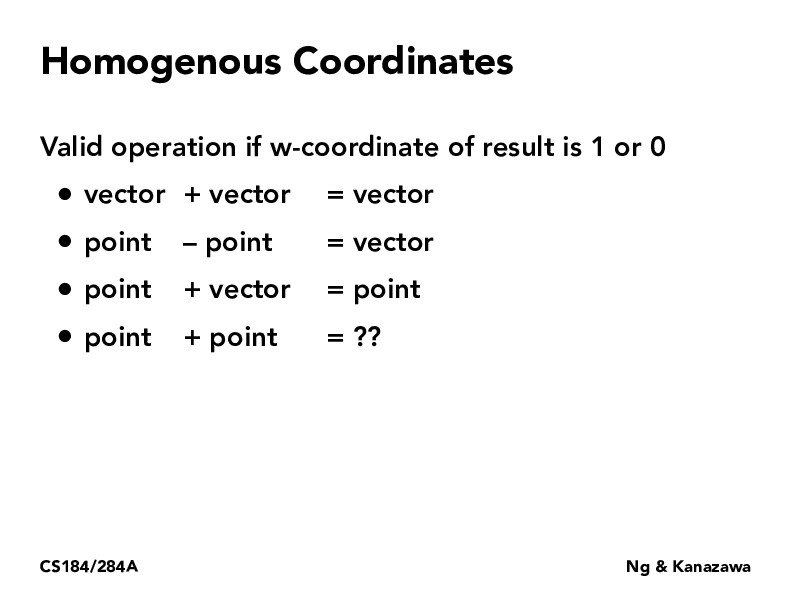Lecture 4: Transforms (24)briana-jin-zhang

I am confused why point - point is valid but point + point is not. Isn't point A - point B = A + (-B)?

Is it related to what it says about w-coord being 1 or 0? Because point + point then becomes w = 2, but point - point means w = 0, which is valid?briana-jin-zhang

I am also confused why w must be 1 because I asked a GSI during discussion if w' could be not 1 and he said yes, so long as x = x'/w' and y = y'/w'ziyicui2022

Hey Briana, this is how I approach this. Point is a "location" or "position", vector is "the direction & distance one walks towards"; a vector can be defined as "the direction & distance one walks from one point to another", that is point - point; but the meaning of (point + point) is undefined here.

You must be enrolled in the course to comment